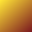#-32768 .. 32767

The memory and data paths often place limits on the range of numbers. Languages have some freedom to reinterpret this limits but rarely go far from expectations.

Early binary machine designs chose word sizes of 12, 16, 18, 24, 32, 60 or 64 bits. Larger words might be divided into bytes of say 8 or 12 bits. See also Decimal Machines.

Current convention has designs of 8, 16, 32 and 64 bit words divided into 8-bit bytes. Machines still vary in how bytes are organized within words with the two most self-consistent variations known as big-endian and little-endian. See Byte Sex.

Each additional bit doubles the range of possible numbers. A 16-bit word can represent 2¹⁶ numbers which will be divided between positives, negatives and zero.

With less than 24 bits one must always be careful to be sure desired numbers won't exceed the available range.

With more than 24 bits one must be dealing with huge numbers or doing unusual things with them to be concerned.

When the range of possible numbers includes negatives then convention has the range of possible numbers divided into two halves with one half, the positive half, shorted by one in the extreme to make room for zero. Hence the range of 16-bit numbers is from -2¹⁵ to 2¹⁵-1 which is -32768 .. 32767. See Complement Notation.

Arithmetic can lead to results that exceed the permissible range of numbers. Often this leads to discarding the most-significant bits such that the result "wraps-around" the permissible number space. See Overflow.

Adding two numbers may require one more bit to represent the result.

Negating a number may require one more bit the represent the result. (i.e. the largest negative number will overflow if negated.)

Multiplying two numbers may require twice as many bits to represent the result.

Some languages support unlimited precision arithmetic where additional words are automatically allocated with results overflow their current representation. See Bignum.

Some languages (hardware) supports "saturated" results where overflow leads to the largest possible result. See Digital Signal Processing.